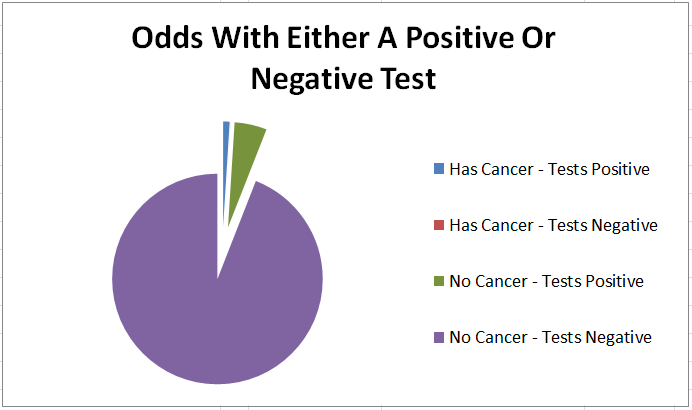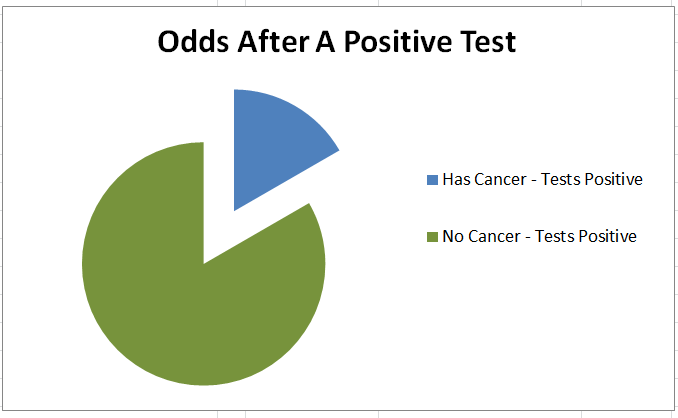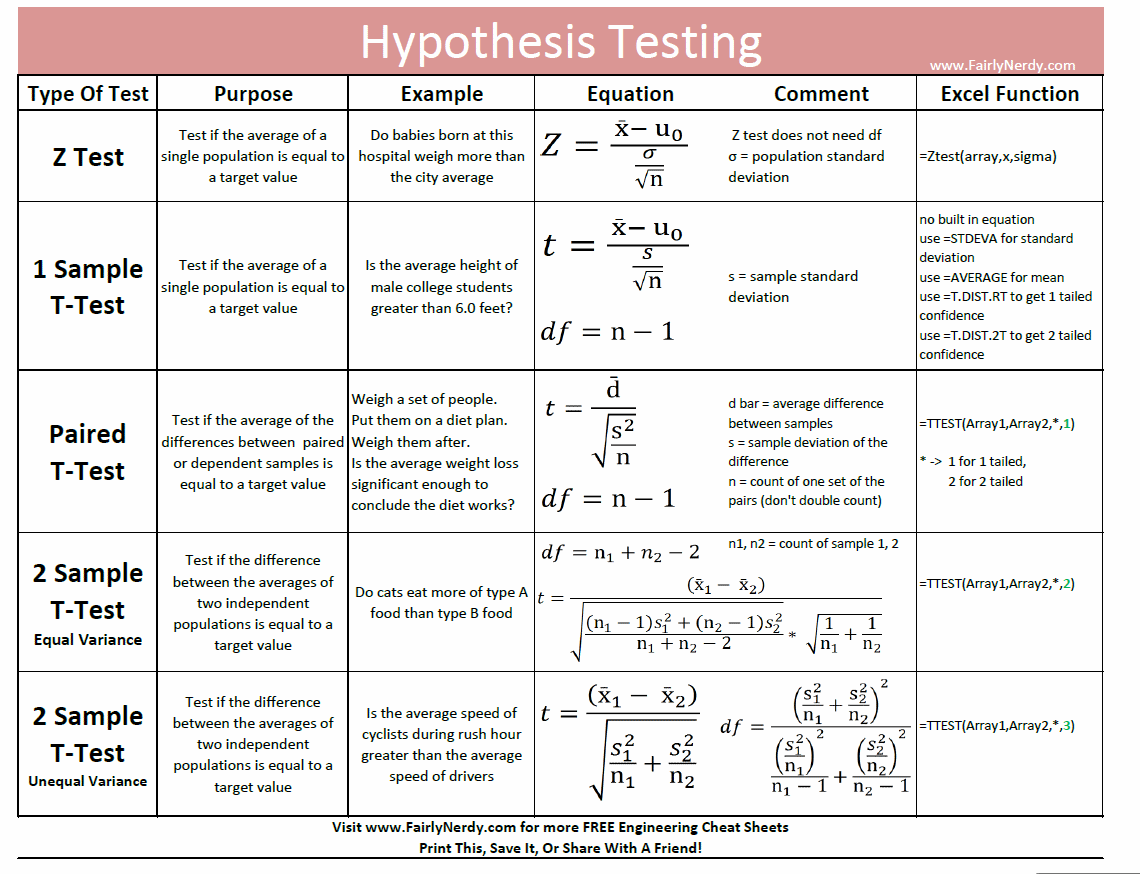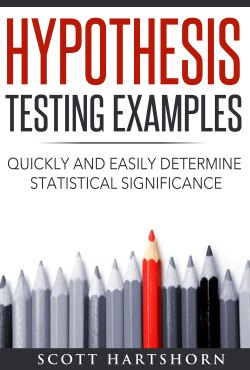# An Intuitive Guide To Bayes Theorem

The purpose of this page is to give you an intuitive understanding of how to solve Bayes Theorem problems.  The equation for Bayes Theorem is not all that clear, but Bayes Theorem itself is very intuitive.  The basics of Bayes Theorem are this

• Everything starts out with an initial probability – That is, before you do any tests or have any data, there is some initial probability of an event
• Tests can update that probability –  After you assign an initial probability, if you gather more information that is relevant then the probability can change.  For instance, you may initially have a very low chance of having an illness, but if a test for that illness comes back positive, the probability that you have it has increased
• After a test, all probabilities get normalized to 1 –  It doesn’t matter if an event is unlikely to have occurred.  What matters is if the event is likely compared to all other possible events.   For instance, if you don’t know whether you are observing a 6 sided die or a 20 sided die, and you see the die roll 4 five times in a row, it is unlikely that the 6 sided die would have rolled those values.  But it is extremely unlikely the 20 sided die would have, so comparatively the 6 sided die is more likely

## 6 Easy Steps For Any Bayes Problem

1. Determine what you want the probabilities for, and what you are observing
2. Estimate initial probabilities for all possible answers
3. For each of the initial possible answers, assume that it is true and calculate the probability of getting the observation with that possibility being true
4. Multiply the initial probabilities (Step 2) by the probabilities based on the observation (Step 3) for each of the initial possible answers
5. Normalize the results
6. Repeat steps 2-5 over and over for each new observation

## Bayes Theorem Applied To Cancer Testing

Testing for a disease is a classic Bayes Theorem problem, and one that can give counter intuitive results the first time you see it.   Let’s say that you are testing a generic patient for cancer.   One percent of the population has this cancer.   You have a test that will return a True Positive (return a positive when they actually do have cancer) 99% of the time, and return a True Negative (return a negative when they do not have cancer) 95% of the time.

You do 1 test, and get back a positive result.   What are the odds this patient actually does have this cancer ?

1. Determine Possibilities

There are two possibilities.  The patient either has cancer.  Or they do not have cancer

2. Estimate Initial Probabilities

Since  this is a generic patient they should be like the general population, so we assume there is a 1% chance they have cancer, and a 99% chance they do not.3. Calculate The Probability Of Getting The Result For Each Possible Answer

The result is a positive test.   If the patient has cancer, the probability of getting that result (True Positive) is 99%.   If the patient does not have cancer, the probability of getting that result (False Positive) is 5%  (which is 1 minus the 95% true negative rate)

4. Multiply Step 2 By Step 3 To Get The Combined Probability

This step should be similar to any other probability you have studied.  We are just calculating what is the probability they have cancer, and got a positive test.  And separately calculating what is the probability they do not have cancer, and got a positive test.5. Normalize The Results

This will be the final answer after 1 positive result.  At this step we see how likely the having cancer was, considering that a false positive was a possibilityAnd that is the answer, we have found that after the “99% Reliable” test, there is only a 16.7% chance that the patient has cancer

6. Repeat The Steps Over Again With Additional Observations

If you do additional tests, you use the new values as your starting probability.  In this case let’s assume that we do a second test, get a Positive result, and then a third test and get a Negative Result.

For the second test, the conditional equation is the same as the first test.  The normalized has cancer value of 16.7% gets multiplied by .99, and the normalized does not have cancer value of 83.33% gets multiplied by .05.

For the third test, since this was a negative result we need to change the formula.  We multiply the normalized has cancer probability by the False Negative rate of .01  (1-.99) , and the normalized does not have cancer rate by the True Negative rate of .95.

The results after both tests are shown below

##After the second positive result, the odds the patient actually has cancer jumps up to 79.8%, but after the negative test, the odds drop back down to 4%

## That Example Was Great, But You Promised Me Intuition

The page promised you intuition.  So far we have solved one Bayes Theorem problem, which is decent example, but not too different than what is on Wikipedia for Bayes Theorem.  Here is the intuition you should develop

• Bayes Theorem Is Just Multiplication and Division –  Bayes theorem itself is very simple.  Multiply out all of the strings of probabilities, and then normalize.  However some problems it is applied to are themselves very complicated, so the whole thing becomes complicated.  For instance, you can make the problem more difficult by using complicated probability distributions for the conditional probabilities or complicated initial probability distribution functions.  There might be special probability functions applied to a goal scoring problem in soccer, or a line waiting problem at a store.  But that doesn’t mean Bayes Theorem itself is all that complicated, the Bayes part of the problem is still just multiplication and division
• It is just as easy to solve for all possibilities as a single one – You might encounter a problem such as “This bag has 4 sided, 6 sided, 8 sided and 12 sided dice in it.  Your friend draws out a die, rolls it, and reports the number as 5.  What is the probability the selected die was a 6 sided die”  The problem asks you to solve for the 6 sided die, but since you have to get the total probability at each step any way in order to normalize, it is just as easy to solve the problem for the 4, 6, 8, and 12 sided dice at the same time.  Solving them all at the same time makes the thought process more straightforward, and can be done in a nice clean table.
• The order of observations doesn’t matter to end results – Bayes theorem amounts to repeated multiplication.  Multiplication is commutative.  You can change the order of the terms and get the same final results.   But if you change the order of the observations, for instance putting the negative cancer test result first in our example problem, the intermediate results will have different probabilities
• You Don’t Actually Have To Normalize Each Step – We normalized the example problem each step.  You could do all of the multiplication for the observations, and then normalize at the end and get the same result.  The only caution is that the probabilities can get very small after repeated decimal multiplication if you do not normalize. You can run into trouble with round off or truncation error depending on what you are using to do the math.

## So Why Was The Cancer Problem Result Surprising ?

Many people are surprised to see that a positive result on the 99% reliable test still only means there was a 16.7% chance the patient had cancer.  Why was that surprising ?  Because most people do not bake the initial probabilities into their intuition.

We do a good job of understanding the conditional probability.  After all, a 99% reliable test should make it much more likely the patient has cancer, which it does.  But if the initial probability is a really small number, the new probability will probably be small as well.  This often gets overlooked, and people implicitly assume an evenly distributed initial probability when thinking about these types of problems.

Overlooking the initial probability is the real joke behind this XKCD comic https://xkcd.com/1132/   (not having to pay the bet if the sun actually exploded is merely a bonus)

These pie charts are a good way to visualize what is occurring.  If the patient doesn’t do any test, the odds of having cancer are a small slice of the pieWhile the patient is waiting for the test results there are 4 possibilities, either they have cancer and the test comes back positive (blue),  they don’t have cancer and the test comes back positive (green),  they don’t have cancer and the test comes back negative (purple)  or they have cancer and the test comes back negative (red slice, but too small to be seen)Once they get positive test results, the purple and red slices of the previous chart go away.   We normalize the green and blue slices in light of the new total probability. The odds of getting that result due to a false positive (green) are still larger than the odds of a true positive (blue).## More Examples

If you want more examples and information about Bayes Theorem, here is a book I wrote walking through half a dozen Bayes Theorem examplesAnd here is an Excel file solution to some Bayes Theorem problems

# Understanding Statistical Significance

## Statistical Significance in Real Life

Statistical significance is a way of quantifying how unlikely something that you are measuring is, given what you know about the baseline.   Exactly how unlikely something needs to be before it is statistically significantly depends on the context.  You likely have an intuitive understanding of statistical significance based on your own life.

For instance,  if you were at a United States airport, and it was announced that your plane was 15 minutes late, you wouldn’t think that it was anything unusual.  But if you were at a Japanese bullet train station, and found out that it was going to be 15 minutes late, you would probably think that was at least somewhat odd.

Why does one seem like a more significant event than the other ?   It is because you know that planes are frequently late, where the trains almost never are.   So the trains being late is more significant because it is more different than the normal day to day variation than the plane being late.

## Plot The Delay

Statistical Significance is very easy to understand on a probability density plot.   The red line shows 15 minutes late.   The blue line shows how likely a train will be any given time late, and the green line shows how likely a plane will be any given time late.   The total area under each of the blue and green lines is 1

It is clear on the chart that very few trains are more than 15 minutes late, but  a lot of planes are.There are really two things going on in the chart.  The first is that the average plane is more late than the average train is.   The average plane is 10 minutes late, and the average train is 0 minutes late.   So being 15 minutes late is bigger difference from average than for a train than a plane.

The second thing that is going on is that the distribution of plane lateness is a lot wider than the distribution of train lateness.  There is a lot more variation in the plane departure time than there is in the train departure time.   Because of that the plane lateness would have to be even greater to be unusual

## The Gist of Statistical Significance

Statistical Significance means quantifying the probability of how unlikely an event is.  Exactly what is statistically significant depends on context, but typical numbers considered statistically significant are if something would have less than a 5% chance, less than a 1% chance, or less than a .5% chance of occurring if there wasn’t some difference between what you are measuring and the baseline.

The information that is important to statistical significance are

• How many measurements you have – The more measurements you have, the more likely you have measured the full population of what is occurring, and not just a non-representative sample
• How different the average of your measurements is from the expected average  –  The bigger the difference, the more likely it is significant.
• How much variation there is in the measurements.  –   The less variation there is in the measurements, i.e. the tighter the spread is, the smaller the difference needs to be to be significant

There are small differences in the equations based on exactly what has been measured, but essentially all of the equations boil down to

• Get a number which is the difference in average values, multiplied by the square root of the number of measurements you have, and divided by the square root of the variation in your measures.   Call that number the “Test Statistic”
• The larger the Test Statistic the more statistically significant the difference.
• Look up the Test Statistic in the appropriate “Z-Table” or “T-Table” to find the probability that there is a statistically significant difference between your samples, as opposed to just random variation

## Equations For Statistical Significance

Now that you have a general understanding of Statistical Significance, it is time to look at the equations.   The most commonly used test for statistical significance is the Z-Test.   You use this test if you have a lot of measurements  (at least 20, preferably at least 40) and you are comparing it against a population with known values.   For example, you would use this test if you work at a hospital that had 500 babies born in it the past year, and you wanted to see if the average weight of those babies was different than the average weight of every baby born in your city.Where

• X_bar   : is the average of the measured data
• U_0      : is the population average
• Sigma  : is the population standard deviation
• n           : is the number of measured samples

You then look up the Z-value in a Z-Table to get probability

There are a few other different equations for Statistical significance called “T-Tests”.   You would use one of these T-Tests instead of a Z-test for one of these reasons

• The number of measurements you have is small, certainly you would use a T-Test with fewer than 20 measurements, or maybe fewer than 50
• You want to compare before and after measurements for the same individual.  For instance, if you have a before and after measurement for 20 people after a diet, you would use a certain type of T-Test.

## What is the difference between a Z-Test and a T-Test?

What is a T-test vs a Z-test, and how do you know when to use a Z-test or a T-test?  The thing to understand about T-Tests, is that they are almost the same as the Z-Test, and will give almost the same answer as you increase the number of measurements that you have.  The whole point of T-Tests is that they put more area at the tail of the normal curve, instead of the middle to account for uncertainty you would have in your measured mean and standard deviation if you have a very small sample size.   Once you get above 20 or so measurements the difference between Z-test results and T-test results becomes vanishingly small.

The plots below show the probability density for a Z-curve, and T-test curves with different sample sizesOnce you get past 20 or so measurements (green line, hardly visible) there really isn’t much of a difference between a T-Test or a Z-test (purple line).  However if you only have a few measurements than the T-Test results will need a lot greater Test Statistic to give a statistically significant result

It can be a little bit confusing knowing exactly which test to use, but using the exact right test isn’t that important unless you are taking an exam or righting a scientific paper.  The tests will all give similar results assuming you have more than 10 measurements, and very similar assuming you have 30 or more.

For a better understanding of the different types of tests, you can refer to this cheat sheet I put together giving the formulas for each test, and when they are used.## Examples of Z-Test vs T-Tests

This post was intending to give an intuitive understanding of statistical significance.   If you are interested in looking at examples of Z-Tests and T-tests and exactly how they are used and in what circumstance you might use one or the other, you can find some examples in this book I’ve put on AmazonOr you can get an Excel file with different hypothesis testing examples here.